MATLAB Function Referencebessely

Bessel functions of the second kind

Syntax

• ```Y = bessely(nu,Z)
Y = bessely(nu,Z,1)
[Y,ierr] = bessely(nu,Z)
```

Definition

The differential equation

•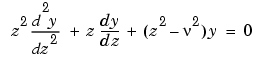where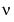is a real constant, is called Bessel's equation, and its solutions are known as Bessel functions.

A solution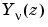of the second kind can be expressed as

•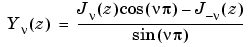where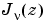and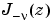form a fundamental set of solutions of Bessel's equation for noninteger•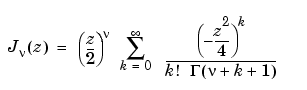and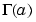is the gamma function.is linearly independent ofcan be computed using `besselj`.

Description

```Y = bessely(nu,Z) ``` computes Bessel functions of the second kind,, for each element of the array `Z`. The order `nu` need not be an integer, but must be real. The argument `Z` can be complex. The result is real where `Z` is positive.

If `nu` and `Z` are arrays of the same size, the result is also that size. If either input is a scalar, it is expanded to the other input's size. If one input is a row vector and the other is a column vector, the result is a two-dimensional table of function values.

```Y = bessely(nu,Z,1) ``` computes `bessely(nu,Z).*exp(-abs(imag(Z)))`.

```[Y,ierr] = bessely(nu,Z) ``` also returns completion flags in an array the same size as `Y`.

 ierr Description `0` `bessely` successfully computed the Bessel function for this element. `1` Illegal arguments. `2` Overflow. Returns `Inf`. `3` Some loss of accuracy in argument reduction. `4` Unacceptable loss of accuracy, `Z` or `nu` too large. `5` No convergence. Returns `NaN`.

Remarks

The Bessel functions are related to the Hankel functions, also called Bessel functions of the third kind,

•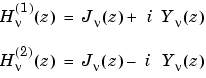where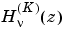is `besselh`,is `besselj`, andis `bessely`. The Hankel functions also form a fundamental set of solutions to Bessel's equation (see `besselh`).

Examples

Example 1.

• ```format long
z = (0:0.2:1)';

bessely(1,z)

ans =
-Inf
-3.32382498811185
-1.78087204427005
-1.26039134717739
-0.97814417668336
-0.78121282130029
```

Example 2. `bessely(3:9,(0:.2:10)')` generates the entire table on page 399 of  Abramowitz and Stegun, Handbook of Mathematical Functions.

Algorithm

The `bessely` function uses a Fortran MEX-file to call a library developed by D. E Amos  .

See Also

`besselh`, `besseli`, `besselj`, `besselk`

References

  Abramowitz, M. and I.A. Stegun, Handbook of Mathematical Functions, National Bureau of Standards, Applied Math. Series #55, Dover Publications, 1965, sections 9.1.1, 9.1.89 and 9.12, formulas 9.1.10 and 9.2.5.

  Carrier, Krook, and Pearson, Functions of a Complex Variable: Theory and Technique, Hod Books, 1983, section 5.5.

  Amos, D. E., "A Subroutine Package for Bessel Functions of a Complex Argument and Nonnegative Order," Sandia National Laboratory Report, SAND85-1018, May, 1985.

  Amos, D. E., "A Portable Package for Bessel Functions of a Complex Argument and Nonnegative Order," Trans. Math. Software, 1986.

© 1994-2005 The MathWorks, Inc.